This is one of the 100+ free recipes of the IPython Cookbook, Second Edition, by Cyrille Rossant, a guide to numerical computing and data science in the Jupyter Notebook. The ebook and printed book are available for purchase at Packt Publishing.

▶  Text on GitHub with a CC-BY-NC-ND license
▶  Code on GitHub with a MIT license

Bokeh (http://bokeh.pydata.org/en/latest/) is a library for creating rich interactive visualizations in a browser. Plots are designed in Python, and they are rendered in the browser.

In this recipe, we will give a few examples of interactive Bokeh figures in the Jupyter Notebook. We will also introduce HoloViews which provides a high-level API for bokeh and other plotting libraries.

Bokeh should be installed by default in Anaconda, but you can also install it manually by typing `conda install bokeh` in a terminal.

To install HoloViews, type `conda install -c ioam holoviews`.

## How to do it...

1.  Let's import NumPy and Bokeh. We need to call `output_notebook()` to tell Bokeh to render plots in the Jupyter Notebook.

```import numpy as np
import pandas as pd
import bokeh
import bokeh.plotting as bkh
bkh.output_notebook()
```

2.  Let's create a scatter plot of random data:

```f = bkh.figure(width=600, height=400)
f.circle(np.random.randn(1000),
np.random.randn(1000),
size=np.random.uniform(2, 10, 1000),
alpha=.5)
bkh.show(f)
```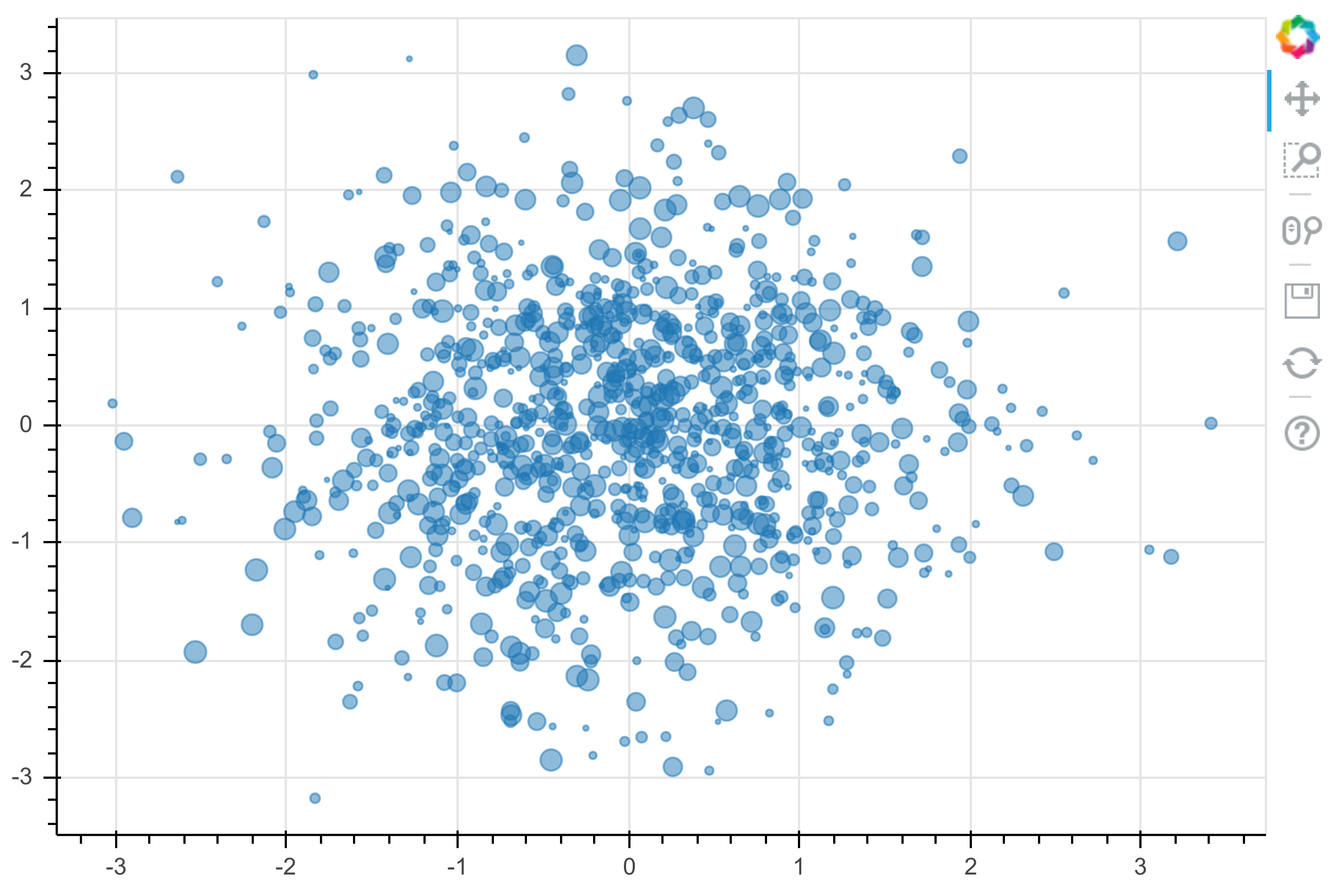An interactive plot is rendered in the notebook. We can pan and zoom by clicking on the toolbar buttons on the right.

3.  Let's load a sample dataset, `sea_surface_temperature`:

```from bokeh.sampledata import sea_surface_temperature
data = sea_surface_temperature.sea_surface_temperature
data
```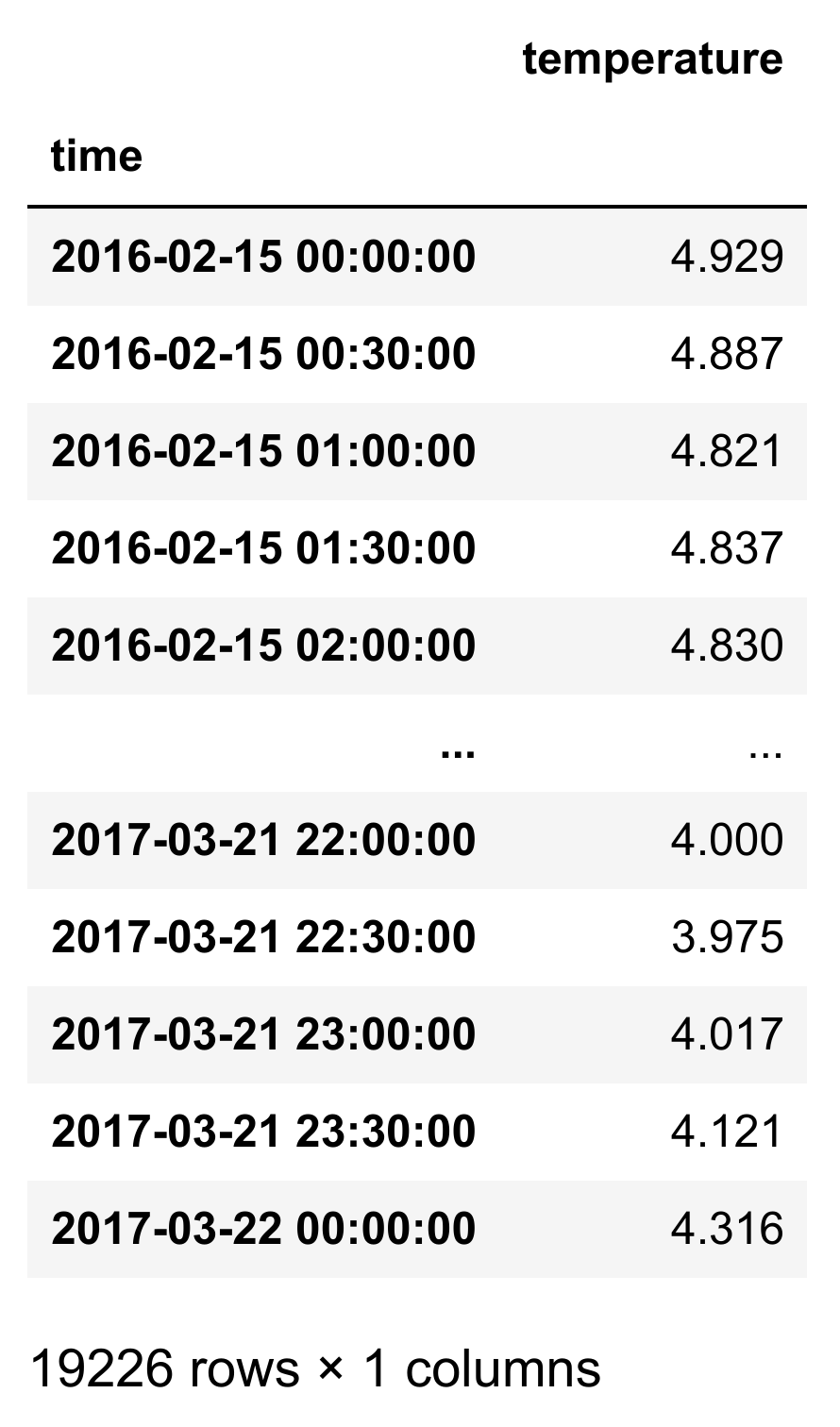4.  Now, we plot the evolution of the temperature as a function of time:

```f = bkh.figure(x_axis_type="datetime",
title="Sea surface temperature",
width=600, height=400)
f.line(data.index, data.temperature)
f.xaxis.axis_label = "Date"
f.yaxis.axis_label = "Temperature"
bkh.show(f)
```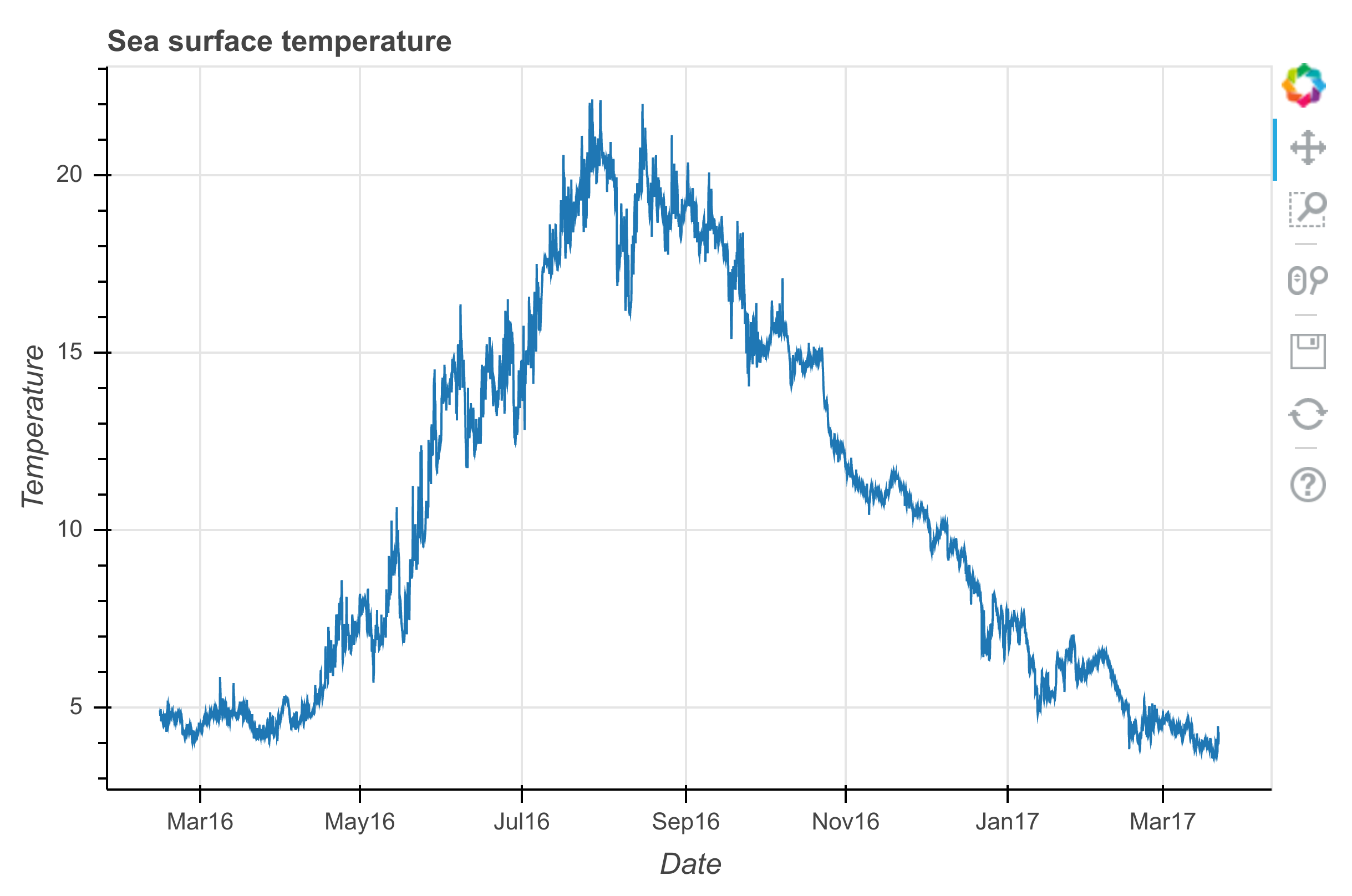5.  We use pandas to plot the hourly average temperature:

```months = (6, 7, 8)
data_list = [data[data.index.month == m]
for m in months]
```
```# We group by the hour of the measure:
data_avg = [d.groupby(d.index.hour).mean()
for d in data_list]
```
```f = bkh.figure(width=600, height=400,
title="Hourly average sea temperature")
for d, c, m in zip(data_avg,
bokeh.palettes.Inferno,
('June', 'July', 'August')):
f.line(d.index, d.temperature,
line_width=5,
line_color=c,
legend=m,
)
f.xaxis.axis_label = "Hour"
f.yaxis.axis_label = "Average temperature"
f.legend.location = 'center_right'
bkh.show(f)
```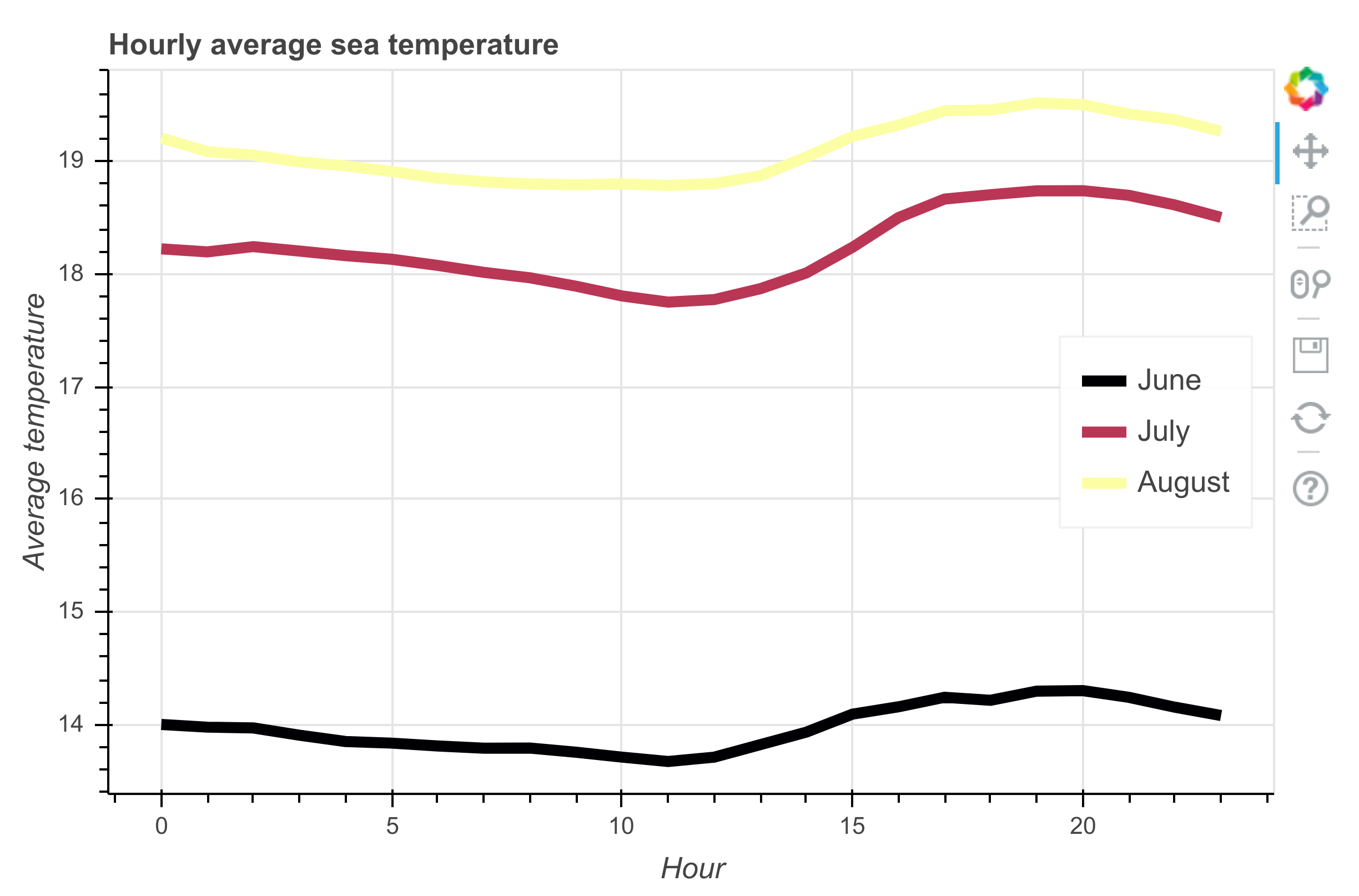6.  Let's move to HoloViews:

```import holoviews as hv
hv.extension('bokeh')
```

7.  We create a 3D array which could represent a time-dependent 2D image:

```data = np.random.rand(100, 100, 10)
```
```ds = hv.Dataset((np.arange(10),
np.linspace(0., 1., 100),
np.linspace(0., 1., 100),
data),
kdims=['time', 'y', 'x'],
vdims=['z'])
```
```ds
```
```:Dataset   [time,y,x]   (z)
```

The `ds` object is a `Dataset` instance representing our time-dependent data. The kdims are the key dimensions (time and space) whereas the vdims are the quantities of interest (here, a scalar `z`). In other words, the kdims represent the axes of the 3D array `data`, whereas the vdims represent the values stored in the array.

8.  We can easily display the 2D image with a slider to change the time, and a histogram of `z` as a function of time:

```%opts Image(cmap='viridis')
ds.to(hv.Image, ['x', 'y']).hist()
```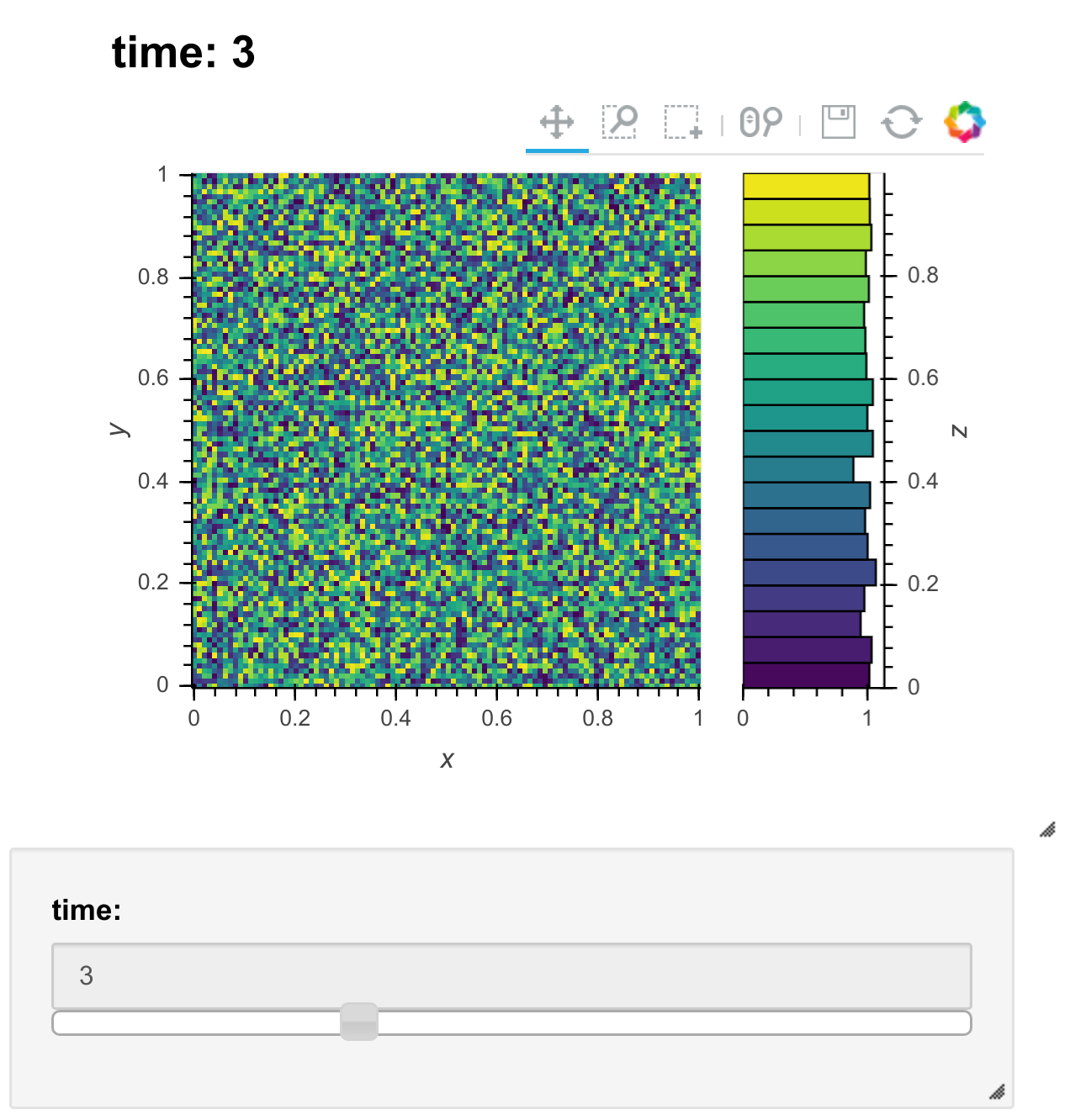## There's more...

Bokeh figures in the Notebook are interactive even in the absence of a Python server. For example, our figures can be interactive in nbviewer. Bokeh can also generate standalone HTML/JavaScript documents from our plots. More examples can be found in the gallery.

The xarray library (see http://xarray.pydata.org/en/stable/) provides a way to represent multidimensional arrays with axes. HoloViews can work with xarray objects.

plotly is a company specialized in interactive visualization. They provide an open-source Python visualization library (see https://plot.ly/python/). They also propose tools for building dashboard-style Web-based interfaces (see https://plot.ly/products/dash/).